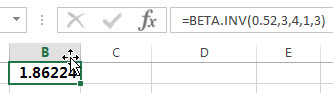# Excel BETA.INV function

This post will guide you how to use the BETA.INV function with syntax and examples in Microsoft excel.

### Description

The Excel BETA.INV function returns the inverse of the beta cumulative probability density function. So if the probability is equal to BETA.DIST(x,…TRUE), then the BETA.INV(probability,…) will return x.

The BETA.INV function is a build-in function in Microsoft Excel and it is categorized as a Statistical Function.

The BETA.INV function is available in Excel 2016, Excel 2013, Excel 2010, Excel 2011 for Mac.

### Syntax

The syntax of the BETA.INV function is as below:

`= BETA.INV(probability, alpha, beta, [A], [B])`

Where the BETA.INV function arguments are:

• Probability -This is a required argument.  A probability associated with the beta distribution, for which you wish to find the value of x.
• Alpha – This is a required argument.  It is a parameter of the distribution.
• Beta – This is a required argument.  It is a parameter of the distribution.
• A – This is an optional argument. A lower bound to the interval of x. If it is omitted, it will be set the default value 0.
• B – This is an optional argument. A upper bound to the interval of x. If it is omitted, it will be set the default value 1.

Note:

• If any argument is non-numeric, the BETA.INV function will return the #VALUE! Error.
• If the Alpha or Beta argument is smaller than or equal to 0, then the BETA.INV function will return #NUM! Error.
• If the argument probability is small than or equal to 0 or greater than 1, the BETA.INV function will Return #NUM! Error.

### Excel BETA.INV Function Examples

The below examples will show you how to use Excel BETA.INV Function to calculate the cumulative beta distribution or beta probability density function.

1# to calculate the inverse of the cumulative beta probability density function for a supplied set of parameters, using the following formula:

`=BETA.INV(0.52,3,4,1,3)`### Related Functions

• Excel BETA.DIST function
The Excel BETA.DIST function can be used to calculate the cumulative beta distribution or beta probability density function.The syntax of the BETA.DIST function is as below:= BETA.DIST(x, alpha, beta, cumulative, [A], [B]).…